Next: Gravitational potential energy Up: Orbital motion Previous: Historical background

## Gravity

There is one important question which we have avoided discussing until now. Why do objects fall towards the surface of the Earth? The ancient Greeks had a very simple answer to this question. According to Aristotle, all objects have a natural tendency to fall towards the centre of the Universe. Since the centre of the Earth coincides with the centre of the Universe, all objects also tend to fall towards the Earth's surface. So, an ancient Greek might ask, why do the planets not fall towards the Earth? Well, according to Aristotle, the planets are embedded in crystal spheres which rotate with them whilst holding them in place in the firmament. Unfortunately, Ptolemy seriously undermined this explanation by shifting the Earth slightly from the centre of the Universe. However, the coup de grace was delivered by Copernicus, who converted the Earth into just another planet orbiting the Sun.

So, why do objects fall towards the surface of the Earth? The first person, after Aristotle, to seriously consider this question was Sir Isaac Newton. Since the Earth is not located in a special place in the Universe, Newton reasoned, objects must be attracted toward the Earth itself. Moreover, since the Earth is just another planet, objects must be attracted towards other planets as well. In fact, all objects must exert a force of attraction on all other objects in the Universe. What intrinsic property of objects causes them to exert this attractive force--which Newton termed gravity--on other objects? Newton decided that the crucial property was mass. After much thought, he was eventually able to formulate his famous law of universal gravitation:

Every particle in the Universe attracts every other particle with a force directly proportional to the product of their masses and inversely proportional to the square of the distance between them. The direction of the force is along the line joining the particles.
Incidentally, Newton adopted an inverse square law because he knew that this was the only type of force law which was consistent with Kepler's third law of planetary motion.

Consider two point objects of masses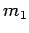and, separated by a distance. As illustrated in Fig. 104, the magnitude of the force of attraction between these objects is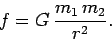(541)

The direction of the force is along the line joining the two objects.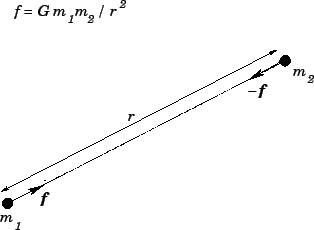Letandbe the vector positions of the two objects, respectively. The vector gravitational force exerted by object 2 on object 1 can be written(542)

Likewise, the vector gravitational force exerted by object 1 on object 2 takes the form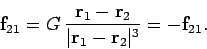(543)

The constant of proportionality,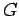, appearing in the above formulae is called the gravitational constant. Newton could only estimate the value of this quantity, which was first directly measured by Henry Cavendish in 1798. The modern value ofis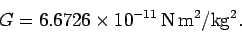(544)

Note that the gravitational constant is numerically extremely small. This implies that gravity is an intrinsically weak force. In fact, gravity usually only becomes significant if at least one of the masses involved is of astronomical dimensions (e.g., it is a planet, or a star).

Let us use Newton's law of gravity to account for the Earth's surface gravity. Consider an object of massclose to the surface of the Earth, whose mass and radius are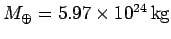and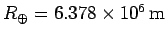, respectively. Newton proved, after considerable effort, that the gravitational force exerted by a spherical body (outside that body) is the same as that exerted by an equivalent point mass located at the body's centre. Hence, the gravitational force exerted by the Earth on the object in question is of magnitude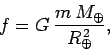(545)

and is directed towards the centre of the Earth. It follows that the equation of motion of the object can be written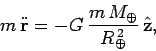(546)

where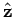is a unit vector pointing straight upwards (i.e., away from the Earth's centre). Canceling the factoron either side of the above equation, we obtain(547)

where(548)

Thus, we conclude that all objects on the Earth's surface, irrespective of their mass, accelerate straight down (i.e., towards the Earth's centre) with a constant acceleration of. This estimate for the acceleration due to gravity is slightly off the conventional value ofbecause the Earth is actually not quite spherical.

Since Newton's law of gravitation is universal, we immediately conclude that any spherical body of mass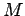and radiuspossesses a surface gravitygiven by the following formula:(549)

Table 6 shows the surface gravity of various bodies in the Solar System, estimated using the above expression. It can be seen that the surface gravity of the Moon is only about one fifth of that of the Earth. No wonder Apollo astronauts were able to jump so far on the Moon's surface! Prospective Mars colonists should note that they will only weigh about a third of their terrestrial weight on Mars.

Table 6: The mass,, radius,, and surface gravity,, of various bodies in the Solar System. All quantities are expressed as fractions of the corresponding terrestrial quantity.
 Body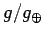Sun109.0 28.1 Moon 0.0123 0.273 0.17 Mercury 0.0553 0.383 0.38 Venus 0.816 0.949 0.91 Earth 1.000 1.000 1.000 Mars 0.108 0.533 0.38 Jupiter 318.3 11.21 2.5 Saturn 95.14 9.45 1.07Next: Gravitational potential energy Up: Orbital motion Previous: Historical background
Richard Fitzpatrick 2006-02-02